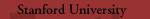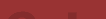Search  | People  | Calendar  | Internal Resources  | Home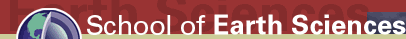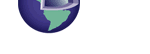# Crustal Deformation and Fault Mechanics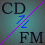## Software

#### hmmvp

Construct an H-matrix and compute matrix-vector products of the form B*x, B(rs,:)*x, and B(rs,cs)*x(cs). An H-matrix is one way to compress the matrix relating slip to stress in a BEM simulation of a fault. The package hmmvpex contains practical usage examples. (Previous major version.)

#### dc3dm

Construct and apply an H-matrix approximation to the displacement-discontinuity method (DDM) linear operator relating quasistatic dislocation and traction components on a nonuniformly discretized rectangular fault in a homogeneous elastic half space.

#### kfgs

Use the adjoint method to compute the gradient of the log likelihood function from a Kalman filter. The gradient calculation takes about as long as running the Kalman filter once independent of the number of parameters.

#### disloc3d

disloc3d is a Matlab wrapper to Y. Okada's DC3.F. It uses geometry conventions specific to our group. There are three versions of the routine: 1. a pure Matlab translation (modified from Coulomb 3.1); 2. a basic Matlab mex wrapper; and 3. a version that (a) parallelizes the calculations using OpenMP and (b) compensates for numerical error that occurs along rays extending from a dislocation element's edges.Last modified Please contact the webmaster with suggestions or comments.